Custom Search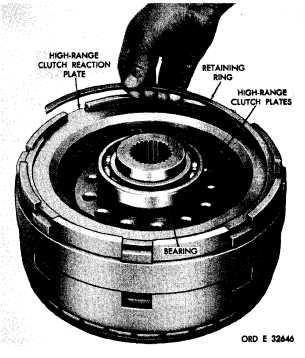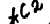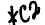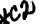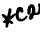*C2 *C2 *C2 *C2 C H A P   5,   SEC  X X V II H I G H     C L U T C H     R E B U I L D PAR  6 - 1 98 .   P l a c e    a    s p a c e r    2 1    o r    2 4    a n d    t h r u st washer   20   or   25   over   the   other   end   of   alining t o o l .    R e m o v e    a l i n i n g    t o o l. .   Slide   the   pinion,   with   its   related   parts into   the   location   in   the   carrier   18   from   which it   was   removed.   Using   alining   tool   8351208, aline   the   pinion,   rollers,   spacers   and   washers. Refer   to   fig.   221. Remove   the   alining   tool. the f o r .   I n s t a l l    s p i n d l e    r e p l a c e r    8 3 5 1 2 6 6    on planetary   carrier   and   position   the   spindle installation   (fig.   226). N o t e .    B e    s u r e    t h a t    t h e    s l o t    o n    t he spindle   is   indexed   properly   with   its lock   pin   bore   in   the   carrier   (fig.   226). i With a press and spindle replacer 8351266,   install   the   planetary   carrier   spindle (fig.   226).   Press   in   the   spindle   to   within   0.010 inch   of   the   carrier   surface. j.   Install   a   lock   pin,   using   a   hammer   and punch   (fig.   224).   Drive   the   pin   0.030   to   0.060 b e l o w    t h e    a d j a c e n t    c a r r i e r    s u r f a c e .    S t a ke metal   over   the   pin. .   I n s t a l l    t h e    r e m a i n i n g    t h r e e    s p i n d l es 19   (fig.   376,   fold-out   5)   and   pinions   22,   with rollers   23,   spacers   21   and   24,   thrust   washers 20   and   25   and   lock   pins   17   in   the   same   manner as   described   in      through  ,   a b o v e. l.   I n s t a l l    t h e    i n t e r m e d i a t e - r a n g e    p l a n e - tary   carrier   assembly   onto   the   low-range   ring gear.   Install   the   retaining   ring   (fig.   223). Section   XXVII.   HIGH-RANGE   CLUTCH   —   REBUILD 1 9 7 .    D E S C R I P T I O N Refer   to   par.   11   for   the   description   of   the high-range    clutch. 1 9 8 .    D I S A S S E M B L Y    ( f i g .    3 7 5 ,    f o l d - o u t    4) .   All   related   items   not   covered in     through  i,   below,   were   removed from   the   transmission   as   outlined   in par.   75,   steps   126   and   127.   No   fur- t h e r    d i s a s s e m b l y    o f    t h e s e    p a r t s    is r e q u i r e d. .   Remove   the   retaining   ring   that   retains the   high-range   clutch   reaction   plate   (fig.   227). Remove   the   reaction   plate. .   R e m o v e    t h e    h i g h - r a n g e    c l u t c h    p l a t es (fig.    227). .   Using   bearing   puller,   remove   the   bear- ing   from   the   hub   of   the   high-range   clutch   hous- ing   (fig.   228). 1 5 4 Figure  227.  Removing  (or  installing)  retaining  ring  that retains high-range clutch reaction plate